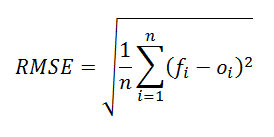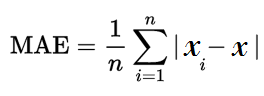Help Center

# Data science: Evaluation measures

The data science questions are evaluated automatically. The solution code in the interface is run in two phases:

• If you click Submit, then your code is evaluated against a private data set (train and test). The final score is assigned based on the private or full data set.

Therefore, your score can change after submitting the solution.

These questions are evaluated by using accuracy measures. The commonly used measures are as follows:

1. Root-mean-square error
2. Mean absolute error

### Root-mean-square error

It is a frequently used measure of the differences between predicted outcomes and observed outcomes. The root-mean-square deviation represents the square root of the second sample moment of the differences between predicted values and observed values or the quadratic mean of these differences. These deviations are called residuals when the calculations are performed over the sample data set and are called errors (or prediction errors) when the computed value is beyond the sample data set. This technique is mainly used in climatology, forecasting, and regression analysis to verify experimental results.

The RMSE formula is as follows:where

• f denotes the expected values
• o denotes the observed values

### Mean absolute error

In this technique, the amount of error in predicted outcomes and observed outcomes. Here, the absolute value of the errors is considered valid for the calculation.

To determine the absolute error (Δx), you must use the following formula:

(Δx) = xi – x

where

• xi denotes the predicted outcome
• x denotes the observed outcome

The mean absolute error (MAE) is the average of all the calculated absolute errors. The formula is:where

• n denotes the number of errors
• Σ (summation symbol) denotes adding all the absolute errors
• |xi – x| denotes the absolute errors